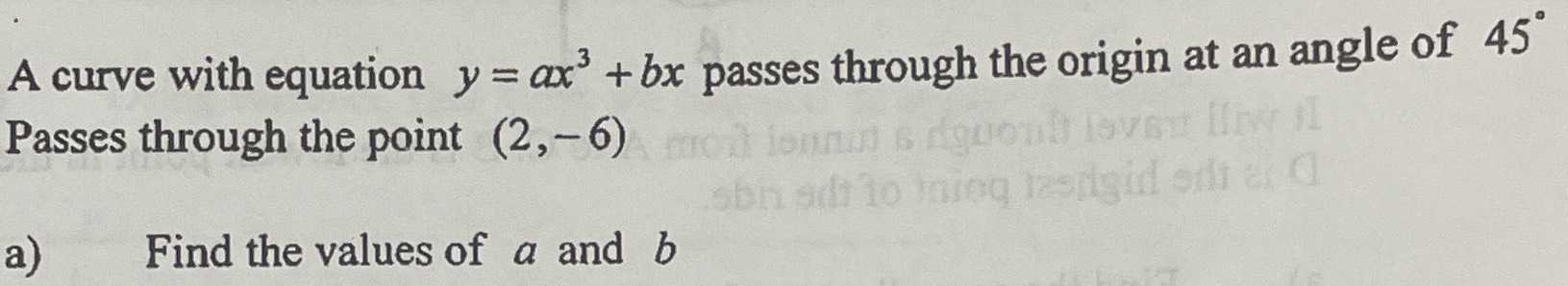Still have math questions?

Calculus
QuestionA curve with equation $$y=ax^{3} +bx$$  passes through the origin at an angle of  $$45°$$

Passes through the point $$( 2 , - 6 )$$

a) Find the values of $$a$$ and $$b$$.

$$a=-1, b=1$$